# Optimal Elliptic Curve Quotients

Let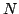be a positive integer and let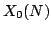be the modular curve over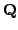that classifies isomorphism classes of elliptic curves with a cyclic subgroup of order. The Hecke algebra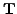of levelis the subring of the ring of endomorphisms ofgenerated by the Hecke operators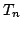for all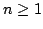. Suppose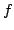is a newform of weight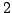for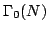with integer Fourier coefficients, and let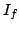be kernel of the homomorphism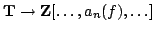that sendsto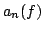. Then the quotient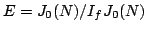is an elliptic curve over. We call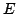the optimal quotient associated to. Composing the embedding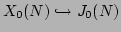that sends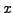to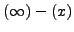with the quotient map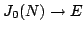, we obtain a surjective morphism of curves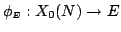. The modular degree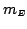ofis the degree of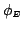.

Let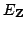denote the Néron model ofover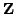. A general reference for Néron models is [BLR90]; for the special case of elliptic curves, see, e.g., [Sil92, App. C, §15], and [Sil94]. Let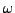be a generator for the rank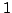-module of invariant differential-forms on. The pullback oftois a differential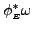on. The newformdefines another differential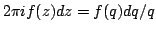on. Because the action of Hecke operators is compatible with the map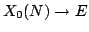, the differentialis a-eigenvector with the same eigenvalues as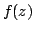, so by [AL70] we have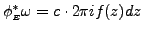for some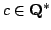(see also [Man72, §5]). The Manin constant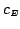ofis the absolute value of the rational number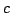defined above.

The following conjecture is implicit in [Man72, §5].

Conjecture 2.1 (Manin)   We have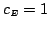.

Significant progress has been made towards this conjecture. In the following theorems,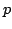denotes a prime anddenotes the conductor of.

Theorem 2.2 (Edixhoven [][Prop. 2)   edix:manin] The constantis an integer.

Edixhoven proved this using an integral-expansion map, whose existence and properties follow from results in [KM85]. We generalize his theorem to quotients of arbitrary dimension in Theorem 3.4.

Theorem 2.3 (Mazur, [][Cor. 4.1)   maziso] If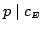, then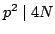.

Mazur proved this by applying theorems of Raynaud about exactness of sequences of differentials, then using the-expansion principle'' in characteristicand a property of the Atkin-Lehner involution. We generalize Mazur's theorem in Corollary 3.7.

The following two results refine the above results at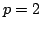.

Theorem 2.4 (Raynaud [][Prop. 3.1)   abbull] If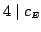, then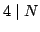.

Theorem 2.5 (Abbes-Ullmo [][Thm. A)   abbull] If, then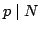.

We generalize Theorem 2.4 in Theorem 3.10. However, it is not clear if Theorem 2.5 generalizes to dimension greater than. It would be fantastic if the theorem could be generalized. It would imply that the Manin constant isfor newform quotients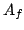of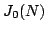, withodd and square free, which be useful for computations regarding the conjecture of Birch and Swinnerton-Dyer.

B. Edixhoven also has unpublished results (see [Edi89]) which assert that the only primes that can divideare,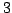,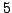, and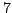; he also gives bounds that are independent ofon the valuations ofat,,, and. His arguments rely on the construction of certain stable integral models for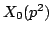.

See Section 5 for more details about the following computation:

Theorem 2.6 (Cremona)   Ifis an optimal elliptic curve overwith conductor at most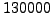, then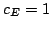.

theorem_type[defi][lem][][definition][][] [Congruence Number] The congruence number tex2html_wrap_inline$r_E$ of tex2html_wrap_inline$E$ is the largest integer tex2html_wrap_inline$r$ such that there exists a cusp form tex2html_wrap_inline$g&isin#in;S_2(&Gamma#Gamma;_0(N))$ that has integer Fourier coefficients, is orthogonal to tex2html_wrap_inline$f$ with respect to the Petersson inner product, and satisfies tex2html_wrap_inline$g &equiv#equiv;f r$. The congruence primes of tex2html_wrap_inline$E$ are the primes that divide tex2html_wrap_inline$r_E$.

To the above list of theorems we add the following:

Theorem 2.7   Ifthen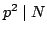or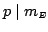.

This theorem is a special case of Theorem 3.11 below. In view of Theorem 2.3, our new contribution is that ifis odd and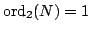, thenis odd. This hypothesis is very stringent--of the optimal elliptic curve quotients of conductor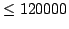, only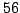of them satisfy the hypothesis. In the notation of [Cre], they are

14a, 46a, 142c, 206a, 302b, 398a, 974c, 1006b, 1454a, 1646a, 1934a, 2606a, 2638b, 3118b, 3214b, 3758d, 4078a, 7054a, 7246c, 11182b, 12398b, 12686c, 13646b, 13934b, 14702c, 16334b, 18254a, 21134a, 21326a, 22318a, 26126a, 31214c, 38158a, 39086a, 40366a, 41774a, 42638a, 45134a, 48878a, 50894b, 53678a, 54286a, 56558f, 58574b, 59918a, 61454b, 63086a, 63694a, 64366b, 64654b, 65294a, 65774b, 71182b, 80942a, 83822a, 93614a

Each of the curves in this list has conductor tex2html_wrap_inline$2p$ with tex2html_wrap_inline$p&equiv#equiv; 34$ prime. The situation is similar to that of [SW04, Conj. 4.2], which suggests there are infinitely many such curves. See also [CE05] for a classification of elliptic curves with odd modular degree.

William Stein 2006-06-25